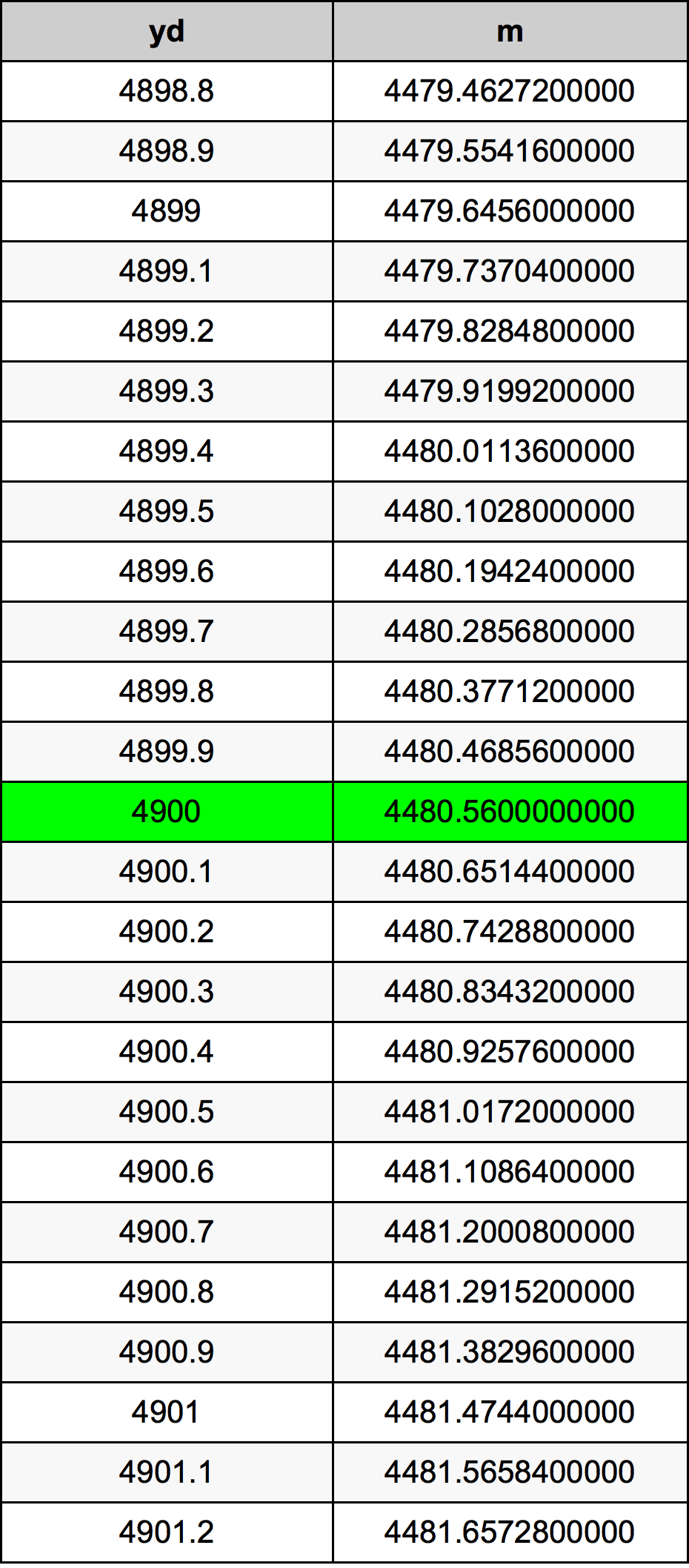Yards To Meters

# 4900 yd to m4900 Yards to Meters

yd
=
m

## How to convert 4900 yards to meters?

 4900 yd * 0.9144 m = 4480.56 m 1 yd
A common question is How many yard in 4900 meter? And the answer is 5358.70516185 yd in 4900 m. Likewise the question how many meter in 4900 yard has the answer of 4480.56 m in 4900 yd.

## How much are 4900 yards in meters?

4900 yards equal 4480.56 meters (4900yd = 4480.56m). Converting 4900 yd to m is easy. Simply use our calculator above, or apply the formula to change the length 4900 yd to m.

## Convert 4900 yd to common lengths

UnitLengths
Nanometer4.48056e+12 nm
Micrometer4480560000.0 µm
Millimeter4480560.0 mm
Centimeter448056.0 cm
Inch176400.0 in
Foot14700.0 ft
Yard4900.0 yd
Meter4480.56 m
Kilometer4.48056 km
Mile2.7840909091 mi
Nautical mile2.4193088553 nmi

## What is 4900 yards in m?

To convert 4900 yd to m multiply the length in yards by 0.9144. The 4900 yd in m formula is [m] = 4900 * 0.9144. Thus, for 4900 yards in meter we get 4480.56 m.

## 4900 Yard Conversion Table## Alternative spelling

4900 Yards to Meters, 4900 Yards in Meters, 4900 Yard to m, 4900 Yard in m, 4900 Yards to Meter, 4900 Yards in Meter, 4900 yd to Meter, 4900 yd in Meter, 4900 Yard to Meter, 4900 Yard in Meter, 4900 yd to m, 4900 yd in m, 4900 Yard to Meters, 4900 Yard in Meters